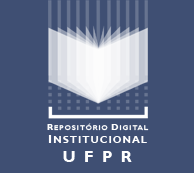# Biblioteca Digitalde Periódicosda Universidade Federal do Paraná

## Open Journal Systems

### NUMERICAL APPROACHES FOR A MATHEMATICAL MODEL OF AN FCCU REGENERATOR

J. C. Penteado, C. O. R. Negrao, L. F. S. Rossi

#### Abstract

This work discusses a mathematical model of an FCCU (Fluid Catalytic Cracking Unit) regenerator. The model assumes that the regenerator is divided into two regions: the freeboard and the dense bed. The latter is composed of a bubble phase and an emulsion phase. Both phases are modeled as a CSTR (Continuously Stirred Tank Reactor) in which ordinary differential equations are employed to represent the conservation of mass, energy and species. In the freeboard, the flow is considered to be onedimensional, and the conservation principles are represented by partial differential equations to describe space and time changes. The main aim ofthis work is to compare two numerical approaches for solving the set of
partial and ordinary differential equations, namely, the fourth-order Runge-Kutta and implicit finite-difference methods. Although both methods give very similar results, the implicit finite-difference method can be much faster. Steady-state results were corroborated by experimental data, and the dynamic results were compared with those in the literature (Han and Chung, 2001b). Finally, an analysis of the model’s sensitivity to the boundary conditions was conducted.

#### Keywords

regenerator; FCC; numerical approaches; finite-difference method; fourth-order Runge-Kutta method

#### Full Text:

PDF

DOI: http://dx.doi.org/10.5380/reterm.v7i1.61745